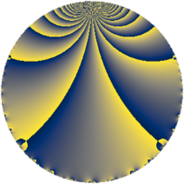# Properties

 Label 48.2.cLevel $48$ Weight $2$ Character orbit 48.c Rep. character $\chi_{48}(47,\cdot)$ Character field $\Q$ Dimension $2$ Newform subspaces $1$ Sturm bound $16$ Trace bound $0$

# Related objects

## Defining parameters

 Level: $$N$$ $$=$$ $$48 = 2^{4} \cdot 3$$ Weight: $$k$$ $$=$$ $$2$$ Character orbit: $$[\chi]$$ $$=$$ 48.c (of order $$2$$ and degree $$1$$) Character conductor: $$\operatorname{cond}(\chi)$$ $$=$$ $$12$$ Character field: $$\Q$$ Newform subspaces: $$1$$ Sturm bound: $$16$$ Trace bound: $$0$$

## Dimensions

The following table gives the dimensions of various subspaces of $$M_{2}(48, [\chi])$$.

Total New Old
Modular forms 14 2 12
Cusp forms 2 2 0
Eisenstein series 12 0 12

## Trace form

 $$2 q - 6 q^{9} + O(q^{10})$$ $$2 q - 6 q^{9} - 4 q^{13} + 12 q^{21} + 10 q^{25} - 20 q^{37} - 10 q^{49} - 12 q^{57} + 28 q^{61} + 20 q^{73} + 18 q^{81} - 36 q^{93} - 28 q^{97} + O(q^{100})$$

## Decomposition of $$S_{2}^{\mathrm{new}}(48, [\chi])$$ into newform subspaces

Label Dim $A$ Field CM Traces $q$-expansion
$a_{2}$ $a_{3}$ $a_{5}$ $a_{7}$
48.2.c.a $2$ $0.383$ $$\Q(\sqrt{-3})$$ $$\Q(\sqrt{-3})$$ $$0$$ $$0$$ $$0$$ $$0$$ $$q-\zeta_{6}q^{3}+2\zeta_{6}q^{7}-3q^{9}-2q^{13}+\cdots$$